# GMAT Math : Calculating the percentage of a sector from an angle

## Example Questions

### Example Question #1 : Calculating The Percentage Of A Sector From An Angle

A sector of a circle has a central angle equal to 45 degrees. What percentage of the circle is comprised by the sector?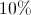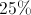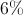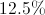Explanation:

The entire circle is 360 degrees, therefore we can set up proportions and cross multiply.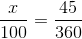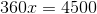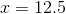### Example Question #2 : Calculating The Percentage Of A Sector From An Angle

Consider the Circle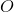: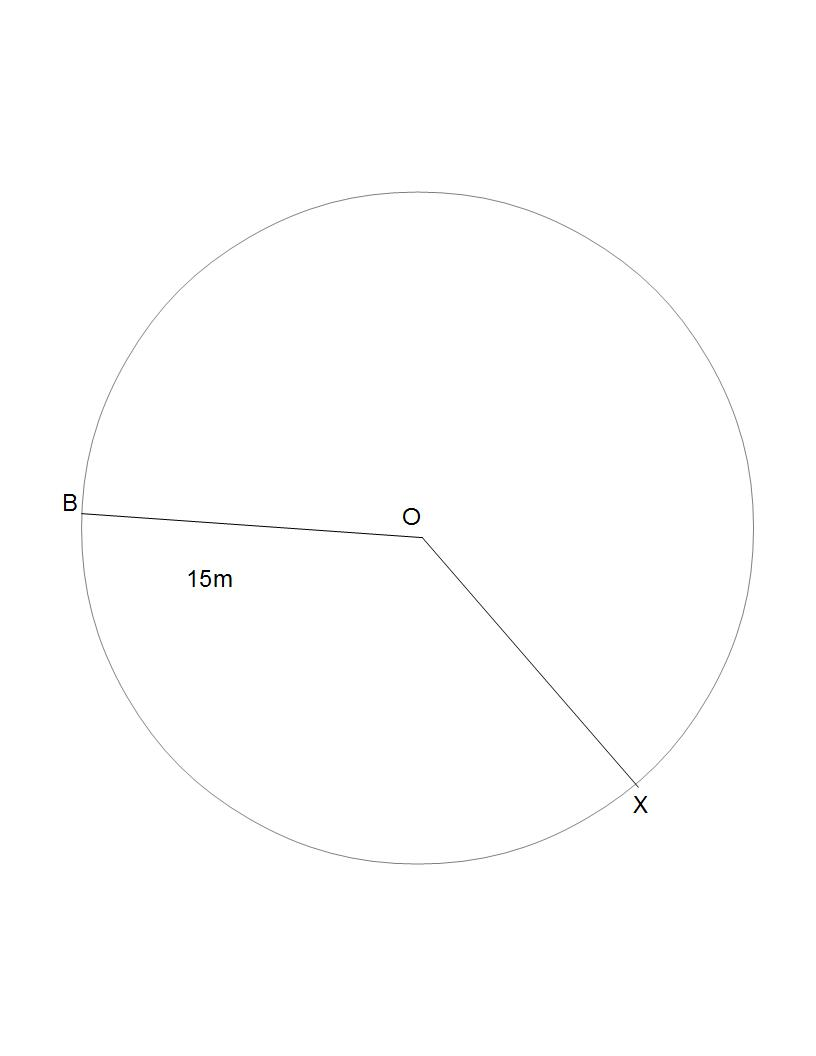(Figure not drawn to scale.)

Suppose sector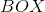covers an area of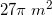. What percentage of the area of the circle does sectorcover?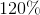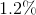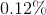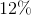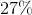Explanation:

To find the percentage of the area of the circle that sectorcovers, divide the area of sectorby the total area of the circle:

Area of the circle: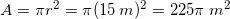Percentage: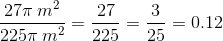To go from a decimal to a percent, multiply by. This gets us to, the correct answer.

### Example Question #3 : Calculating The Percentage Of A Sector From An Angle

Circle T represents a round birthday cake. If the first slice will have a central angle ofdegrees, what percentage of the total cake is in the first slice?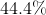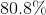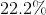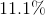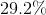Explanation:

In this question, the slice of cake can be thought of as a sector. We are given that its central angle is 80 degrees and asked to find what percentage of the whole it represents. Straightforward division is all we need here. We are not give a radius or any way of finding one. All we need to find is the percentage of the whole. To do that, recall that a circle has 360 degrees total and compute the following: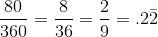From here multiply by 100 to get the percentage.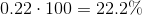So the first slice represents about 22.2% of the total cake!

### Example Question #4 : Calculating The Percentage Of A Sector From An Angle

If a sector has an angle of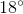, what percentage of the circle's area is covered by the sector?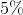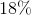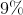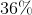Explanation:

The percentage of a circle covered by a sector is equal to the angle of the sector divided by the full measure of the circle,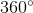. The given sector has an angle of, so whatever percent this is ofwill tell us what percent of the circle's area is covered by the sector: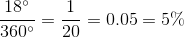### Example Question #5 : Calculating The Percentage Of A Sector From An Angle

What percentage of a circle is a sector if the angle of the sector is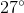?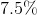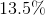Explanation:

The full measure of a circle is, so any sector will cover whatever fraction of the circle that its angle is of. We are given a sector with an angle of, so this sector will cover a percentage of the circle equal to whatever fractionis of. This gives us: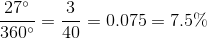### Example Question #6 : Calculating The Percentage Of A Sector From An Angle

What percentage of a circle's total area is covered by a sector with an angle of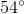?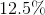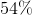Explanation:

The full measure of a circle is, so any sector will cover whatever fraction of the circle that its angle is of. We are given a sector with an angle of, so this sector will cover a percentage of the circle equal to whatever fractionis of. This gives us: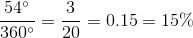Tired of practice problems?

Try live online GMAT prep today.